### Home > CALC > Chapter 10 > Lesson 10.4.2 > Problem10-150

10-150.
1. Find the radius and interval of convergence for each of the following power series. Homework Help ✎

1.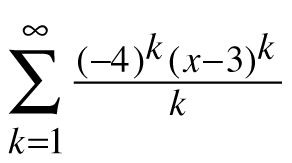2.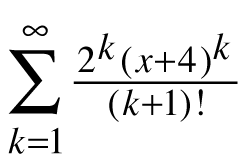3.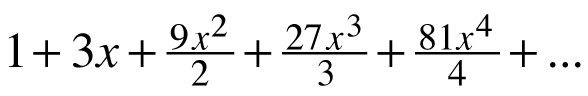4.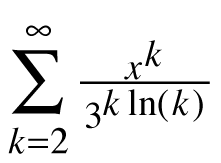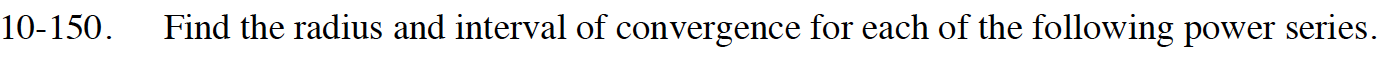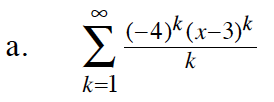$\lim_{k\to\infty}\Big|\frac{(-4)^{k+1}(x-3)^{k+1}}{k+1}\cdot\frac{k}{(-4)^k(x-3)^k}\Big|<1$

$|x-3|\lim_{k\to\infty}\Big|\frac{4k}{k+1}\Big|<1$

$2\frac{3}{4}

Now test the convergence of the series at each endpoint of the interval.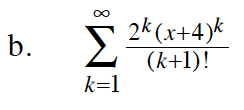$\frac{(k+1)!}{(k+2)!}=\frac{1}{k+2}$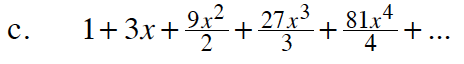$=\sum_{k=0}^\infty \frac{(3x)^k}{k}$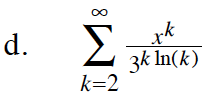After applying the Ratio Test, you should get:

$3|x|<1$

Now test the convergence of the series using the endpoints of the interval.